#### You may also like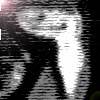### Clock Hands

This investigation explores using different shapes as the hands of the clock. What things occur as the the hands move.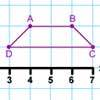### Transformation Tease

What are the coordinates of this shape after it has been transformed in the ways described? Compare these with the original coordinates. What do you notice about the numbers?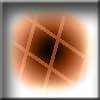### Penta Play

A shape and space game for 2, 3 or 4 players. Be the last person to be able to place a pentomino piece on the playing board.

# Coordinate Challenge

##### Age 7 to 11Challenge Level

Here is a grid: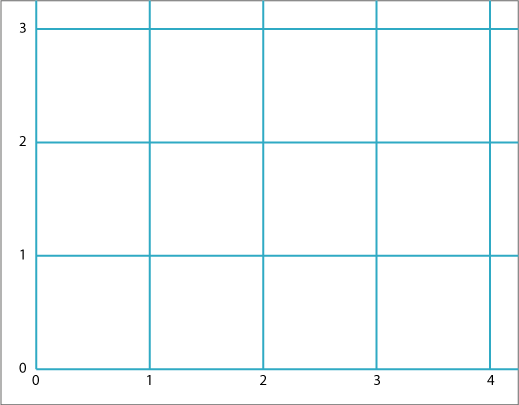Can you position these ten letters in their correct places according to the eight clues below?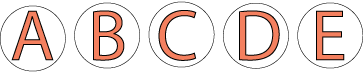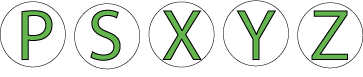Clues:

The letters at $(1, 1),$ $(1, 2)$ and $(1, 3)$ are all symmetrical about a vertical line.

The letter at $(4, 2)$ is not symmetrical in any way.

The letters at $(1, 1),$ $(2, 1)$ and $(3, 1)$ are symmetrical about a horizontal line.

The letters at $(0, 2),$ $(2, 0)$ have rotational symmetry.

The letter at $(3, 1)$ consists of just straight lines.

The letters at $(3, 3)$ and $(2, 0)$ consist of just curved lines.

The letters at $(3, 3),$ $(3, 2)$ and $(3, 1)$ are consecutive in the alphabet.

The letters at $(0, 2)$ and $(1, 2)$ are at the two ends of the alphabet.